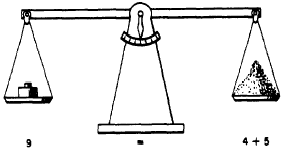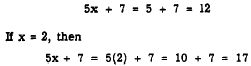Chapter 11 - Linear Equations in one variableCustom SearchCHAPTER 11 LINEAR EQUATIONS IN ONE VARIABLE One of the principal reasons for an intensive study of polynomials, grouping symbols, factoring, and fractions is to prepare for solving equations. The equation is perhaps the most important tool in algebra, and the more skillful the student becomes in working with equations, the greater will be his ease in solving problems. Before learning to solve equations, it is necessary to become familiar with the words used in the discussion of them. An EQUATION is a statement that two expressions are equal in value. Thus,         4+5=9 and         A = lw (Area of a rectangle = length x width) are equations. The part to the left of the equality sign is called the LEFT MEMBER, or first member, of the equation. The part to the right is the RIGHT MEMBER, or second member, of the equation. The members of an equation are sometimes thought of as corresponding to two weights that balance a scale. (See fig. 11-l.) This compari8on is often helpful to students who are learning to solve equations. It is obvious, inFigure 11-l. Equation compared to a balance scale.  the case of the scale, that any change made in one pan must be accompanied by an equal change in the other pan. Otherwise the scale will not balance. Operations on equations are based on the same principle. The members must be kept balanced or the equality is lost. CONSTANTS AND VARIABLES Expressions in algebra consist of constants and variables. A CONSTANT is a quantity whose value remains the same throughout a particular problem. A VARIABLE is a quantity whose value is free to vary. There are two kinds of constants-fixed and arbitrary. Numbers such as 7, -3, 1/2, and p are examples of FIXED constants. Their values never change. In 5x + 7 = 0, the numbers 0, 5, and 7, are fixed constants.  ARBITRARY constants can be assigned different values for different problems. Arbitrary constants are indicated by letters-quite often letters at the beginning of the alphabet such as a, b, c, and d. In ax+b=0,. the letters a and b represent arbitrary constants. The form ax t b - 0 represent many linear equations. If we give a and b particular values, say a - 5 and b = 7, then these constants become fixed, for this particular problem, and the equation becomes 5x t 7 = 0 A variable may have one value or it may have many values in a dlscuseion. The letters at the end of the alphabet, such as x, y, z, and w, usually are used to represent variables. In 5x + 7, the letter x is the variable. If x = 1, thenand so on for as many values of x as we desire to select. If the expression 5x + 7 is set equal to some particular number, say -23, then the resulting equality         5x + 7 = -23 holds true for just one value of x. The value is -6, since         5(-6) + 7 = -23 In an algebraic expression, terms that contain a variable are called VARIABLE TERMS. Terms that do not contain a variable are CONSTANT TERMS. The expression 5x + 7 contains one variable term and one constant term. The variable term is 5x, while 7 is the constant term. In ax + b, ax is the variable term and b is the constant term. A variable term often is designated by naming the variable it contains. In 5x + 7, 5x is the x-term. In ax + by, ax is the x-term, while by is the y-term.Integrated Publishing, Inc. - A (SDVOSB) Service Disabled Veteran Owned Small Business